Learning Objective

In this lesson we will learn how the progress of a chemical reaction can be explained by particle collision theory. We will also learn how to measure the rate of a chemical reaction.

Learning Outcomes

By the end of this lesson you will be able to:

• Define rate of reaction.
• Describe how the rate of a chemical reaction changes as it progresses.
• Explain how the progress of a chemical reaction is related to the frequency of successful particle collisions.
• Measure the instantaneous rate and average rate of a chemical reaction.

Rate of a Chemical Reaction

1 | Chemical Kinetics and Collision Theory

2 | The Progress of a Chemical Reaction

3 | Measuring Instantaneous Rate and Average Rate

4 | Summary

Click images to preview the worksheet for this lesson and the Year 10 Chemistry Workbook (PDF and print versions)

## Chemical Kinetics

• Chemical reactions involve the conversion of one or more reactants into one or more products.
• They can be represented as:• How quickly reactants are converted to products varies for different reactions.
• The speed of a reaction is largely determined by the chemical properties of the reactants, but is also affected by factors such as temperature, concentration of reactants, surface area of reactants and the presence of catalysts.
• Chemical kinetics is the study of chemical reaction rates and the factors that affect them.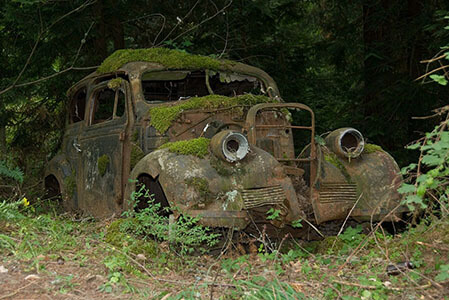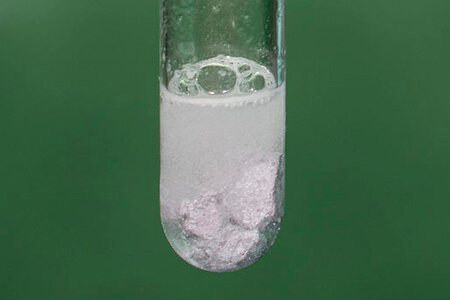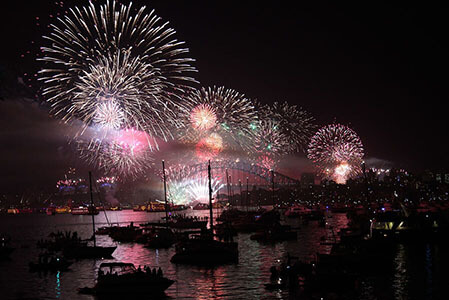Rates of chemical reactions vary widely – they can take place over many years or in a fraction of a second.

(Images: jc112203, Pixabay; Adam Rędzikowski, Wikimedia Commons; kalipsotri, Pixabay)

## Collision Theory

• Collision theory describes the interactions between reactant particles during a chemical reaction.
• It can be used to explain rates of chemical reactions and how certain factors, such as temperature, concentration, surface area and catalysts, affect the rate of a reaction.
• The collision theory states that for a reaction to occur between two reactant particles:
• Reactant particles must collide.
• They must collide with sufficient energy and correct orientation to break bonds.
• Only when these criteria are met will the reactant particles be converted to product particles. These are referred to as ‘successful’ collisions.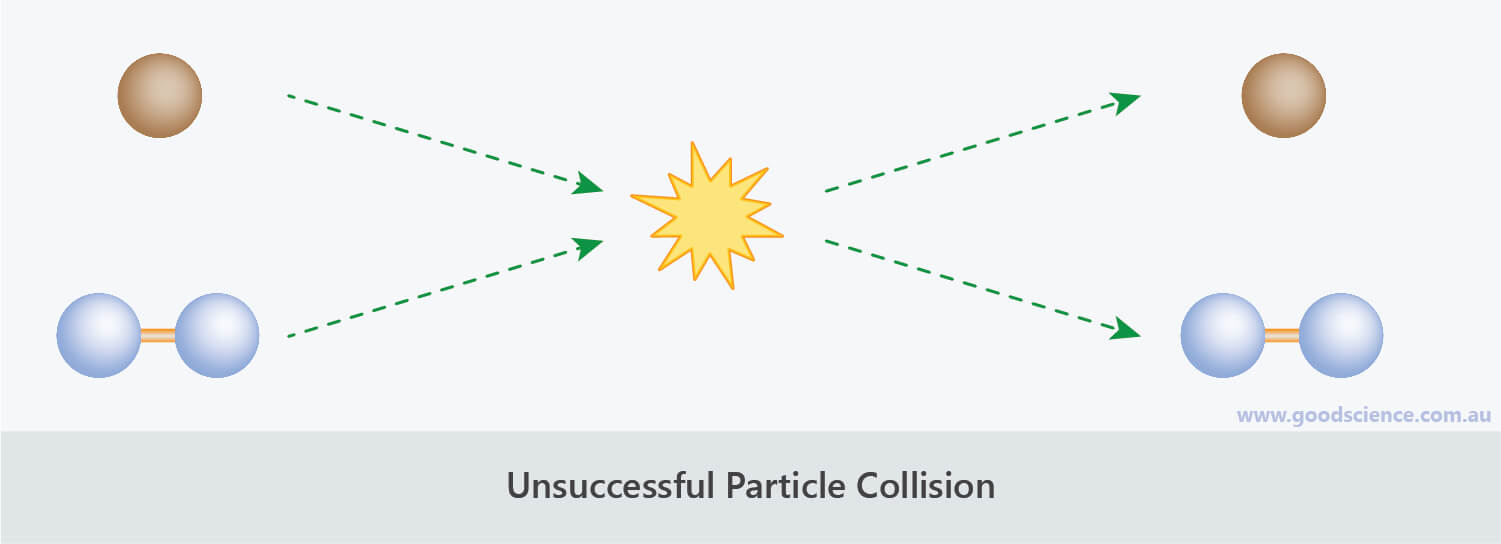To be converted into products, reactant particles must collide with sufficient energy and the correct orientation.

## Reaction Rate

• ‘Rate’ refers to how quickly (or slowly) something happens.
• It is always measured with respect to time.
• The rate of a chemical reaction can be measured as the change in the amount of reactants or products over time.
• The rate of a reaction can therefore be measured in two ways:
• How quickly reactants are consumed.
• How quickly products are formed.
• This can be represented as:• Determining the rate of a chemical reaction will therefore involve measuring the amount of a particular reactant or product at time intervals as the reaction progresses.

## Progress of a Reaction

• Chemical reactions do not proceed at a constant rate.
• There is, however, a pattern to how the rate changes during the course of the reaction:
• Initially, the reaction is at its maximum rate.
• This is because there is the highest concentration of reactant particles, resulting in the highest frequency of collisions between them.
• Then reaction rate decreases as the reaction progresses.
• This is because, as reactants are converted to products, there is a decrease in the concentration of reactant particles, resulting in a decrease in the frequency of collisions between them.
• Eventually, the reaction stops.
• This is because all of the reactants have been converted to products, hence there are no more collisions between reactant particles.

## Graphing the Progress of a Reaction

• If the rate of a reaction was measured by the consumption of reactants, its graph would look like the blue line below.
• If the rate of a reaction was measured by the formation of products, its graph would look like the black line below.
• For any graph, the steeper the slope, the greater the rate of change.
• Both of the graph lines below show that a chemical reaction is at its fastest at the beginning of the reaction, then gradually decreases as the reaction proceeds, until the reaction stops.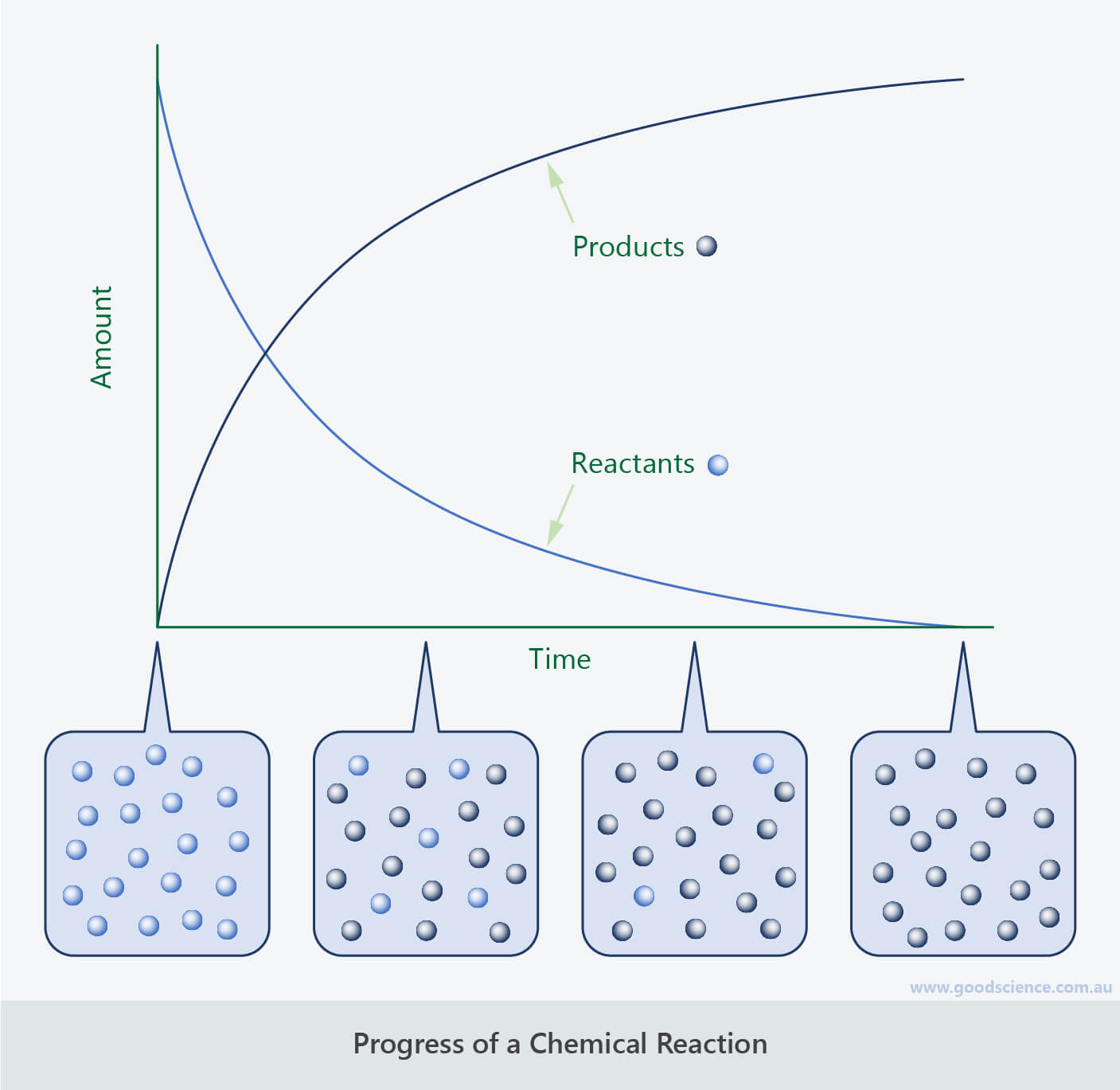The progress of a chemical reaction can be measured in terms of the appearance of products or the disappearance of reactants.

## Instantaneous Rate and Average Rate

• By measuring the change in amount of reactant or product over time, the rate of a reaction can be determined two ways:
• Instantaneous rate – the rate of change at a particular point in time.
• Average rate – the rate of change over a period of time.

## Measuring Instantaneous Reaction Rate

• The instantaneous rate is given by the tangential slope at a given time point.
• For example, for the following reaction, the rate of reaction at 2 minutes is equal to the slope of the curve at 2 minutes.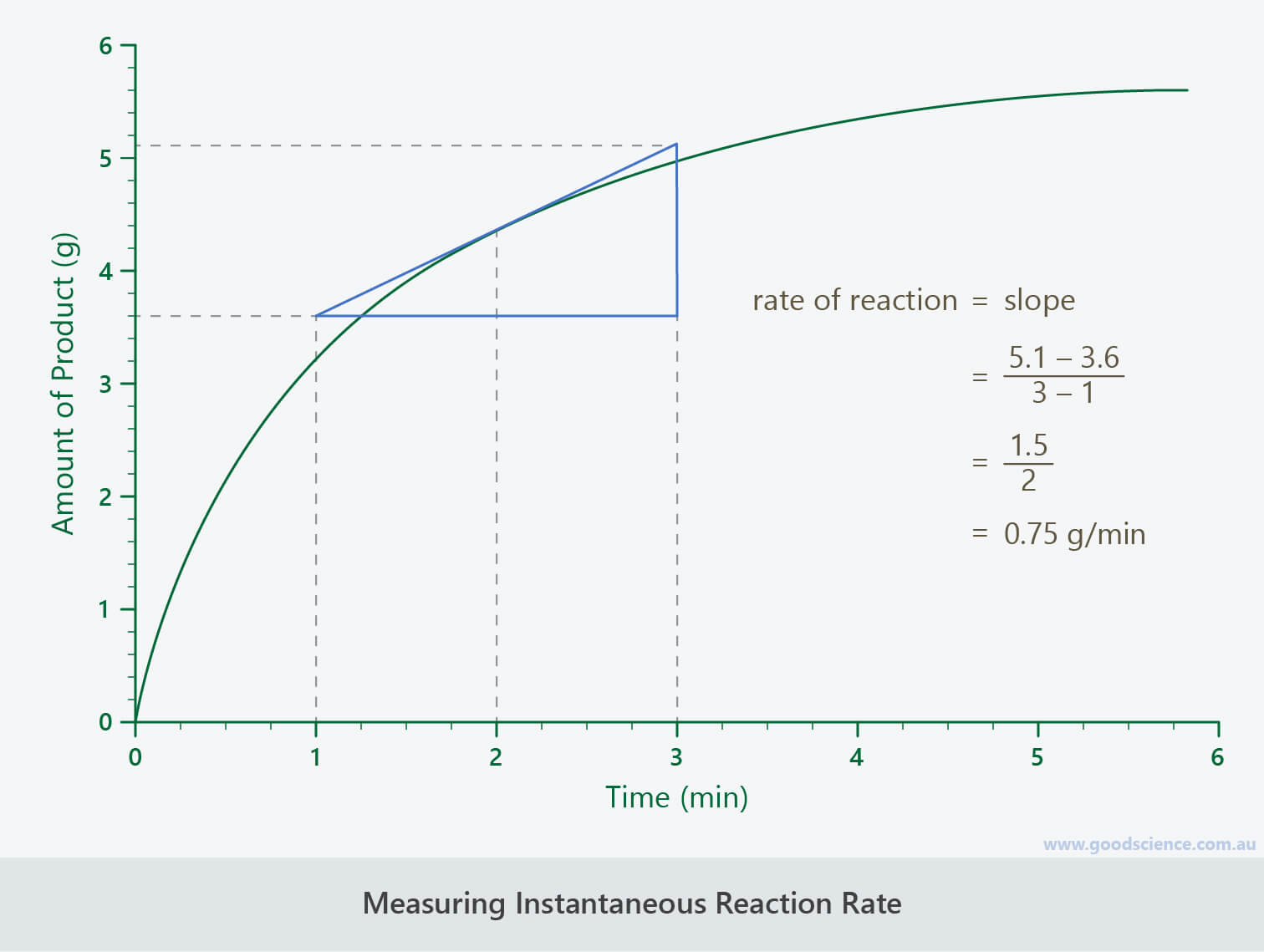Instantaneous reaction rate is the rate of change at a point in time.

## Measuring Average Reaction Rate

• An average reaction rate may cover the entire time period of a chemical reaction, or it may cover any time period during the course of the reaction, such as the first five minutes.
• The average rate is given by the slope between two time points.
• For example, for the following reaction, the average rate of reaction for the first 2 minutes is equal to the change in amount between 0 and 2 minutes.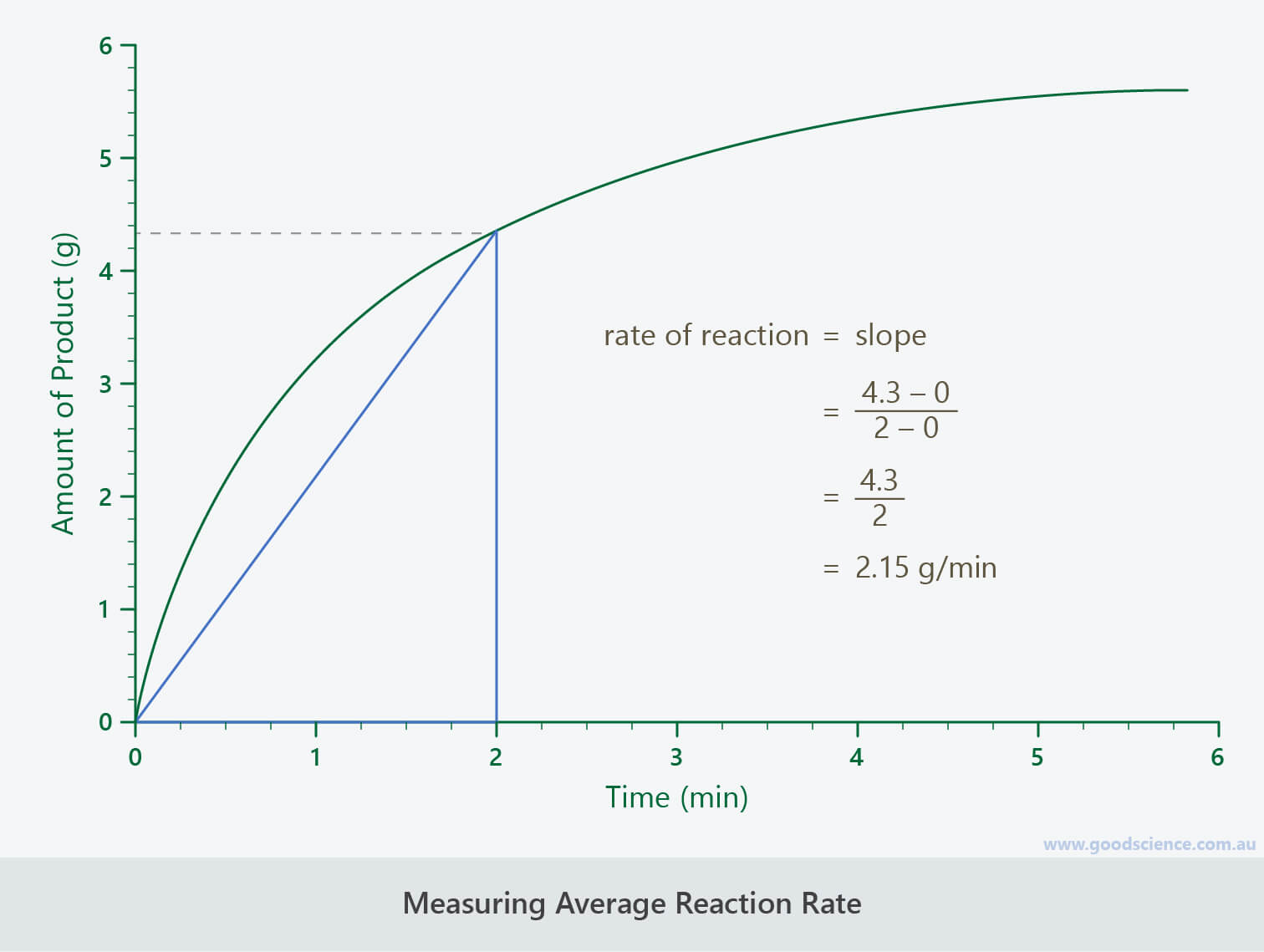Average reaction rate is the rate of change over a period in time.

## Summary

• Collision theory states that for a chemical reaction to occur:
• Reactant particles must collide.
• Reactant particles must collide with sufficient energy and correct orientation to break bonds.
• The rate of a chemical reaction refers to how quickly reactants are converted to products.
• The rate of a reaction can be measured two ways:
• Instantaneous rate – the rate of change at a particular point in time.
• Average rate – the rate of change over a particular period of time.
• Chemical reactions do not proceed at a constant rate.
• Initially, the reaction is at its fastest, as there is the highest concentration of reactant particles, resulting in the highest frequency of collisions between them.
• Rate of reaction = maximum.
• Slope of graph = maximum.
• Concentration of reactants = maximum.
• Concentration of products = 0.
• As a reaction progresses, the rate decreases, as there is a decrease in the concentration of reactant particles, resulting in a decrease in the frequency of collisions between them.
• Rate of reaction decreases.
• Slope of graph decreases.
• Concentration of reactants decreases.
• Concentration of products increases.
• Eventually, the reaction stops, as all of the reactants have been converted to products, hence there are no more collisions between reactant particles.
• Rate of reaction = 0.
• Slope of graph = 0.
• Concentration of reactants = 0.
• Concentration of products = maximum.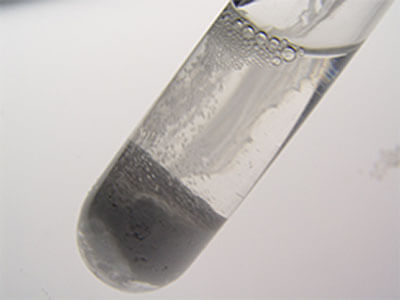(Image: LoyalSoldier, Wikimedia Commons)